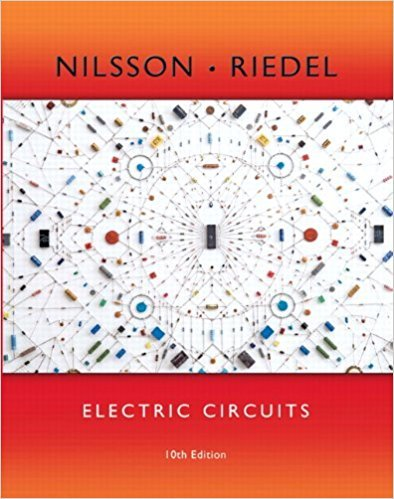×
×

# Answer: a) Use component values from Appendix H to create a first-order RC circuit (seeISBN: 9780133760033 390

## Solution for problem 7.59 Chapter 7

Electric Circuits | 10th Edition

• Textbook Solutions
• 2901 Step-by-step solutions solved by professors and subject experts
• Get 24/7 help from StudySoup virtual teaching assistantsElectric Circuits | 10th Edition

4 5 1 304 Reviews
16
3
Problem 7.59

a) Use component values from Appendix H to create a first-order RC circuit (see Fig. 7.21) with a time constant of 250 ms. Use a single capacitor and a network of resistors, if necessary. Draw your circuit. b) Suppose the capacitor you chose in part (a) has an initial voltage drop of 100 V.At ,a switch connects a current source with a value of 1 mA in parallel with the capacitor and equivalent resistance. Write an expression for the voltage drop across the capacitor for . c) Using your result from part (b), calculate the time at which the voltage drop across the capicitor reaches 50 V.

Step-by-Step Solution:
Step 1 of 3

th STAT 3600 Reference: Chapter 1 of Devore’s 8 Ed. Maghsoodloo Definition. A population is a collection (or an aggregate) of objects or elements that, generally, have at least one characteristic in common. If all the elements can be well defined and placed (or listed) onto a frame from which the sample can be drawn, then the population is said to be...

Step 2 of 3

Step 3 of 3

##### ISBN: 9780133760033

Unlock Textbook Solution